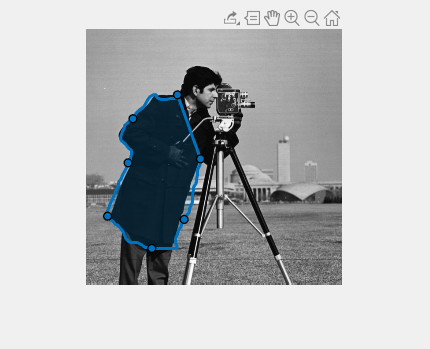# reduce

Package: images.roi

Reduce density of points in ROI

## Syntax

``reduce(ROI)``
``reduce(ROI,tolerance)``

## Description

example

````reduce(ROI)` reduces the number of points that define the region-of-interest `ROI`. The ROI object stores the point array in the `Position` property.`reduce` replaces the original value of the `Position` property with the reduced value.The `reduce` method calls the `reducepoly` function which uses the Douglas-Peucker line simplification algorithm. This algorithm removes points along a straight line and leaves only knickpoints (points where the line curves).```

example

````reduce(ROI,tolerance)` reduces the number of points that define the `ROI`, where `tolerance` specifies the sensitivity of the reduction. Specify the `tolerance` value in the range `[0,1]`.```

## Examples

collapse all

Read an image into the workspace.

`I = imread('cameraman.tif');`

Display the image.

`imshow(I);`

Draw a `Freehand` ROI on the image.

`roi = drawfreehand;`

View the number of points in the `Position` property after completing the shape.

`disp(['Original Size of Position property: ' mat2str(size(roi.Position))]);`
```Original Size of Position property: [272 2] ```

Use the `reduce` object function to reduce the number of points required to define the shape.

`reduce(roi)`View the reduced number of points in the `Position` property.

`disp(['Reduced Size of Position property: ' mat2str(size(roi.Position))]);`
```Reduced Size of Position property: [100 2] ```

Read an image into the workspace.

`I = imread('cameraman.tif');`

Display the image.

`imshow(I);`

Draw a `Polyline` ROI on the image.

`roi = drawpolyline;`

View the number of points in the `Position` property after completing the shape.

`disp(['Original Size of Position property: ' mat2str(size(roi.Position))]);`
```Original Size of Position property: [12 2] ```

Use the `reduce` object function to reduce the number of points required to define the shape.

`reduce(roi)`

View the reduced number of points in the `Position` property.

`disp(['First try at reducing the number of points: ' mat2str(size(roi.Position))]);`
```First try at reducing the number of points: [12 2] ```

`N`ote that the number of points is not changed. To improve the result, change the Tolerance parameter. By default, tolerance is set to .01. Increase the value and try it again.

`reduce(roi,0.3)`View the size of the `Position` property again. Changing the tolerance resulted in a reduction.

`disp(['Reduction after resetting tolerance parameter: ' mat2str(size(roi.Position))]);`
```Reduction after resetting tolerance parameter: [4 2] ```

## Input Arguments

collapse all

ROI object, specified as one of the following ROI objects: `AssistedFreehand`, `Freehand`, `Polygon`, and `Polyline`.

Sensitivity of reduction, specified as a number in the range [0, 1]. Increasing the tolerance increases the number of points removed. A tolerance value of `0` reduces a minimum number of points. A tolerance value of `1` results in the maximum reduction in points, leaving only the end points of the line.

## Algorithms

The Douglas-Peucker line simplification algorithm recursively subdivides a shape looking to replace a run of points with a straight line. The algorithm checks that no point in the run deviates from the straight line by more than the value specified by `tolerance`.